Next: The Binary Independence Model Up: The Probability Ranking Principle Previous: The 1/0 loss case   Contents   Index

## The PRP with retrieval costs

Suppose, instead, that we assume a model of retrieval costs. Let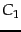be the cost of not retrieving a relevant document and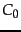the cost of retrieval of a nonrelevant document. Then the Probability Ranking Principle says that if for a specific document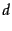and for all documents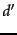not yet retrieved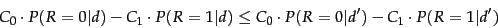(62)

thenis the next document to be retrieved. Such a model gives a formal framework where we can model differential costs of false positives and false negatives and even system performance issues at the modeling stage, rather than simply at the evaluation stage, as we did in Section 8.6 (page). However, we will not further consider loss/utility models in this chapter.